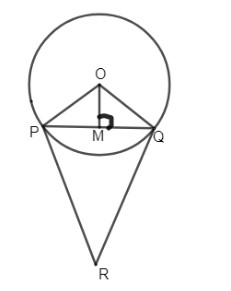Courses
Courses for Kids
Free study material
Free LIVE classes
MoreLIVE
Join Vedantu’s FREE Mastercalss

# If $\angle RPQ={{45}^{\circ }}$, find $\angle PRQ$A. ${{60}^{\circ }}$B. ${{90}^{\circ }}$C. ${{120}^{\circ }}$D. ${{150}^{\circ }}$Verified
360.9k+ views
Hint: Consider, $\vartriangle PQR$, use the properties of the isosceles triangle and find the $\angle PRQ$. PR and QR are tangents from a point R meeting the circle at P and Q.

Given a figure, with a circle whose center is O. From the figure we can say that PR and QR are tangents to the circle from a point R. Three tangents from an external point to the circle are equal.
$\therefore PR=QR$
Now let us consider the isosceles triangle, $\vartriangle PRQ$.
As it is an isosceles triangle, we know that two sides of an isosceles triangle are equal.
In $\vartriangle PRQ$, we know that PR = QR.
Thus the angle RPQ is equal to angle PQR, because they are the base angles of an isosceles triangle.
We have been given, $\angle RPQ={{45}^{\circ }}$.
$\angle RPQ=\angle PQR={{45}^{\circ }}$.
The angle sum property of triangle, states that the sum of interior angles of a triangle is ${{180}^{\circ }}$.
Hence, in the isosceles triangle PRQ, the sum of all the interior angles is ${{180}^{\circ }}$.
$\therefore \angle RPQ+\angle PQR+\angle PRQ={{180}^{\circ }}$
We need to find the $\angle PRQ$.
We already found out that, $\angle RPQ=\angle PQR={{45}^{\circ }}$.
Thus substituting these values in the sum of triangle,
\begin{align} & \angle RPQ+\angle PQR+\angle PRQ={{180}^{\circ }} \\ & {{45}^{\circ }}+{{45}^{\circ }}+\angle PRQ={{180}^{\circ }} \\ & \angle PRQ=180-45-45=180-90 \\ & \angle PRQ={{90}^{\circ }} \\ \end{align}
Hence, we got $\angle PRQ={{90}^{\circ }}$, which makes $\vartriangle PRQ$ right angled at R.
$\therefore$Option (b) is the correct answer.

Note: By seeing the figure, you may try to take $\vartriangle POQ$ instead of $\vartriangle PRQ$, understand the question that we only need to find $\angle PRQ$. So we should take, $\vartriangle PRQ$. As tangents from the external point of the circle are equal, the sides of the triangle are same, thus angles are same and we can get $\angle PRQ$easily.
Last updated date: 29th Sep 2023
Total views: 360.9k
Views today: 7.60k